Neural Network - Perceptron                                 Home : www.sharetechnote.com

Perceptron Fundamentals

As you may know (or sense, feel), it 'sounds' as Neural Network is a kind of algorithm that try to minic the nerve system in biological organism (like human brain). Also as you may know almost every biological organism is made up of basic unit called 'cell'(I used to be a biologist a few decodes ago and as a biologist many things are popping up in my mind that contradicts this statement, but let's not get too much deep into this). Anyway, we (especially scientist or engineers) like to analyze a system as a combination of simple basic units. The basic units of most of biological system is called 'Cell'. In the same logic, when we are talking about Nerve System, it is also made up of a basic unit. This basic unit is called 'Neuron'. If we compare a neural network with biological nerve system, you may easily guess there might be some basic unit that comprises the neural network, that unit is called a Perceptron. The original concep of Perceptron comes from this paper(Ref ), but it is not required to read the paper unless you are seriously interested in the origin of anything.

In this note, I will talk about the simplest Neural Network and how it works. If you are serously interested in Neural Network, you should understand this structure completely and should be able to trace down all the learning process by hand. If you just read the text, you may think you would understand when you read it, but you would get confused 10 minutes after you close the book.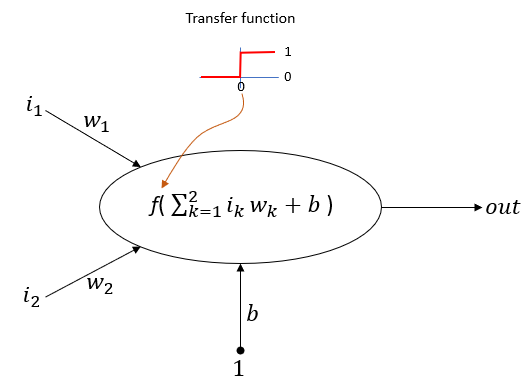NOTE : In the illustration above, the function f() is labeled as Transfer Function. This is called more frequently as Activation Function. In this tutorial, I will use a very simple type of Transfer function called HardLimiter, there are a lot of other types of Transfer Function (Activation Function) and to determine which type of Activation function to use is very important part of neural network construction. Most of neural network software provides various different types of activation function. See Matlab Machine Learning Toolbox Activation function and Pytorch Activation Function to see the diversity of these functions.

There are a few basic things that you MUST understand very clearly. Here goes the list and these are the main things that you will learn from this page by hands.

• How all the inputs are combined to a single value ?
• How the combined value is modified (this modification is done by special function called Actication function or Transfer function).
• How the perceptron learns (this learning is done by a special mechnism called back propagation).

Now let's go through the process of how this network works step by step. I strongly suggest you to draw this network and learning process by pen and pencil on the paper. Each of the process has its own mathematical background that sound scary like matrix inner product, gradient descent etc. But before diving into those mathematics, just blindly follow through these steps and let your brain come up with a clear image in your mind.

Example : Perceptron for AND logic

In this tutorial, I will go through the learning process of the perceptron learning AND gate logic. Actually this is one of the most common example for explaining the logic of the perceptron. In this example, I will show only the first cycles of learning process. In real implementation, you would need to repeat the same process many times until you reach the correct status of the learning process.

I strongly, strongly, strongly recommend you to get sheets of papers and pen, and go through each and every calculation on your own. Even better thing to do is to repeat this cycle several more times and see how each iteration get closer to the final learning states. You can get an example showing 50 iterations of the process from my visual note linked here. Going through at least a few iterations with pen and paper on your own would give you much better understandings than just reading tens of document.

After the completion of the learning process, the newtork should be able to implement following truth table.

 in1 in2 out 1 1 1 1 0 0 0 1 0 0 0 0

Step 1 : Start with an empty network as shown below. This shows the structure of the simplest network (Perceptron) but the values for each component is all empty. Only the learning factor (Alpha) is set to be '1' for simplicity.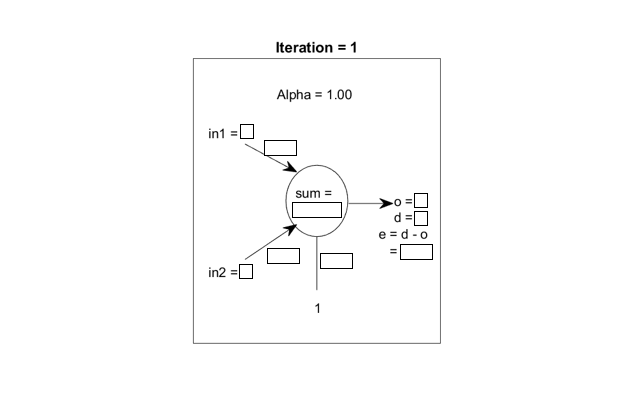Step 2 :  Set all the weight factors (w1, w2, b) with any arbitrary values. In real application of neural network with more complicated structure, determining these initial value can be an important technique... but for many situation you may just put some random value.  In this example, I set the initial value as shown below.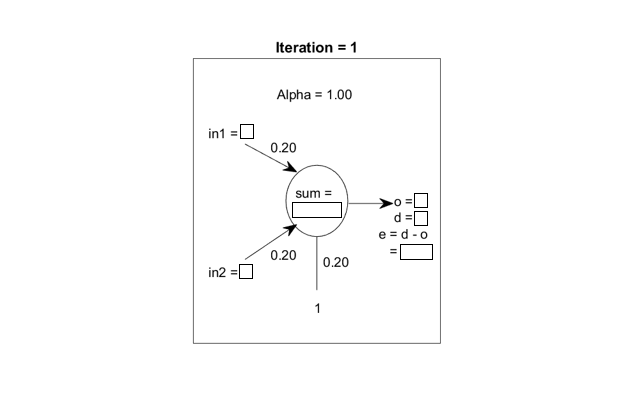Step 3 : Determine the initial training input. Now you can pick up any one set of input from the truth table. Once you pick an any one input set, you automatically knows the desired output (d) from the same table. My initial values are set as follows.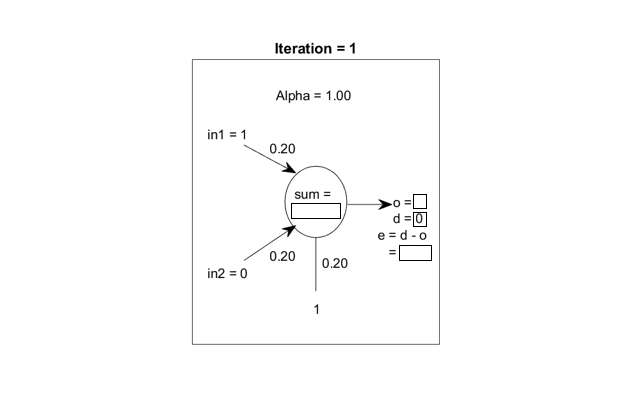Step 4 :  Calculate the output of the network. This step can be split into multiple procedures as shown below.

i) calculate the sum of multiplication of input and weights plus bias value as in step (1)

ii) put the sum into the transfer function of the perceptron. In this case, Hardlimit() is used as the transfer function of the cell (perceptron). Step (2)

iii) the result of the transfer function becomes the output value of the cell (perceptron). Step (3)

iv) calculate the error value. Since we know the output value and desired value, we can calculate the error as in step (4).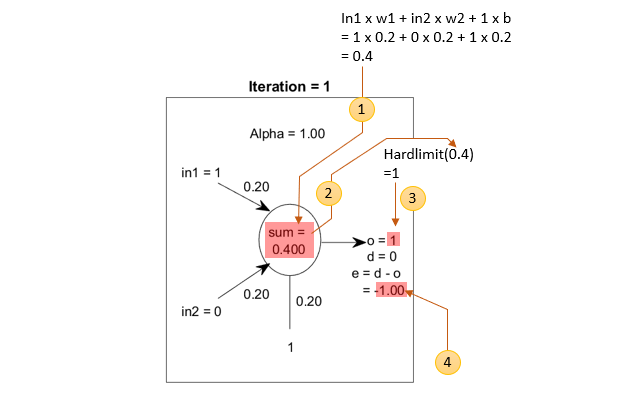Step 5 : Update the Weight and Bias. Once you complete the first iteration as explained above, you can update (change) the weighting value based on the result of this iteration. This parameter updating process is a critical step in neural network and this upading process is called 'Learning'. In perceptron, the learning (weighting update) is done in following way.

Following is to show how the weight value gets updated.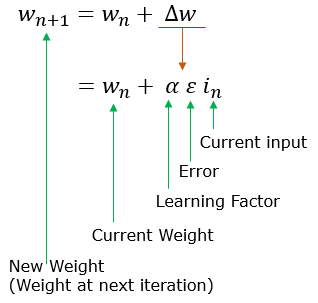Following is to show how the bias value gets updated.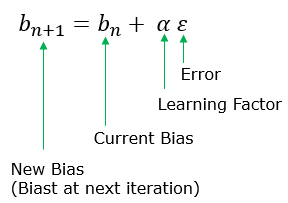You can apply this rule to our example and get the new update as shown below.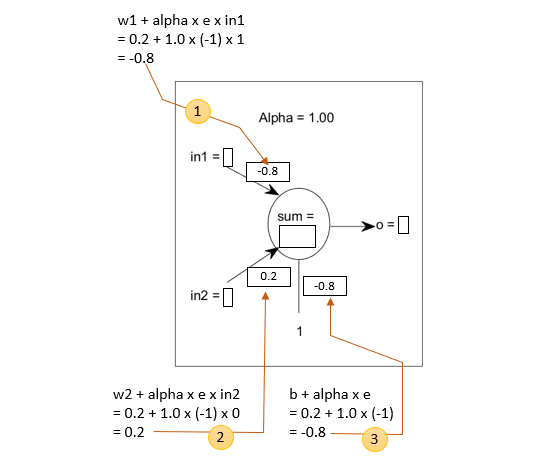Step 6 :  Now it is time to train the network with the new input value. The new input value that I selected is as follows.Step 7 : Now you can estimate the output of the network and error as you did in step 4 and come up with the following result.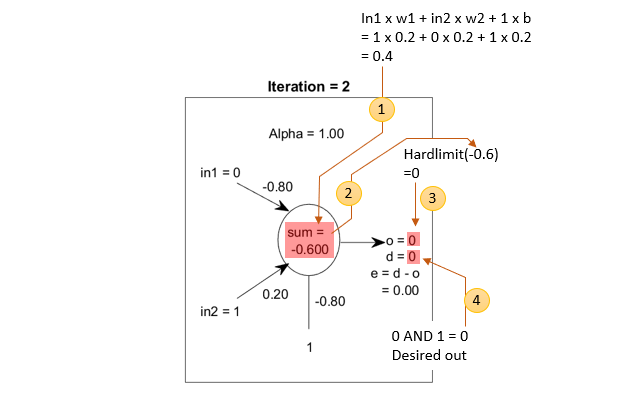Step 8 : Now you can update weight and bias as you did in step 5 and come up with the following result.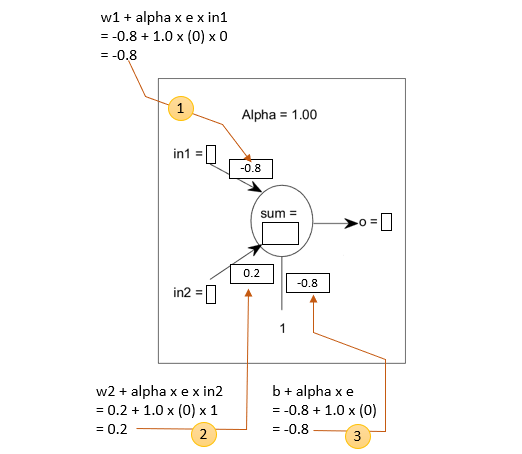I just completed each and every steps of the operation of a single perceptron. If you go through these procedure over and over eventually the network will learn how to come up with the proper outputs for every inputs in AND truth table.

Reference